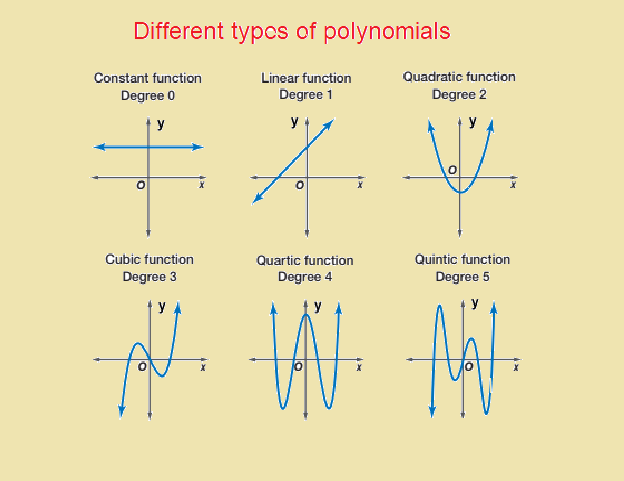September 30, 2023

# Polynomials : Definition, Types of polynomials and Examples, Degree of a polynomial

Polynomials:  An algebraic expression is an expression which is made up of variables and constants along with some algebraic operations.  The several parts of an algebraic expression seperated by + or – operations are called the terms of the  expression. e.g.

(i)   is  an algebraic expression with three terms  and three variables

.

(ii)   is  an algebraic expression with three terms  and two variables .

(iii)    is  an algebraic expression with two terms  and one variable .

(iv)      is  an algebraic expression with one terms  and one variable.

In an algebraic expression , if the powers of variables are non-negative integers , then it is a polynomial.

In the above examples , (i) and (ii) are polynomials, where as (iii) and (iv) are not polynomials.

Contents

# Polynomials in one variable :

Polynomials in one variable are algebraic expressions that consists of  terms in the form of , where  is non-negative integer and a is constant . e.g. all are polynomials  in variable .

Each term of a polynomial has a  coefficient . so in , the  coefficient of is -1, coefficient of is and coefficient of is 3.

## Degree of a polynomial:

The degree of a polynomial in a single  variable is the highest power of in its expression. e.g. is a polyn0mial of degree 5 and is a polynomial of degree 6.

In general  any polynomial of degree is an expression of the form

where    are constants ,    and is a non-negative integer .

Here is called the constant term of the polynomial and are called the coefficient of respectively.

In particular if all the constants are zero , then we get the zero polynomial.  Zero polynomial has no non-zero terms so the degree of zero polynomial is not defined.

### Constant polynomial:

A polynomial containing only the constant term is called constant polynomial. e.g. all are constant polynomials.

Let   is a non-zero constant polynomial ,

then can be written as

Therefore the degree of any non-zero constant polynomial is zero.

Based  on the number of terms,  polynomials are classified as

(i) A polynomial containing one term  is called a monomial. e.g. etc. all are monomials.

(ii) A polynomial containing two terms  is called a binomial. e.g. etc.

(iii)A polynomial containing three terms  is called a trinomial. e.g.  etc. all are trinomials.

#### Linear polynomial :

A polynomial of degree one is called  a linear polynomial. A linear polynomial in is  of the form

.

Any linear polynomials in have  at most two terms . e.g. all are linear polynomials.

A polynomial of degree 2 is called a quadratic polynomial. A quadratic polynomial in one variable will have at most tree terms.  Any quadratic polynomial in will be of the form

###### Cubic polynomial :

A polynomial of  degree  3 is called  cubic polynomials. Any  cubic  polynomial can have at  most 4 terms.  all are examples of cubic polynomials.#### Bina singh

View all posts by Bina singh →

## One thought on “Polynomials : Definition, Types of polynomials and Examples, Degree of a polynomial”

1.Divyanshi says:

Very good explanation & examples

ssc chsl 2023 tier 1 cut off NIRF Rankings 2023 : Top 10 Engineering colleges in India CBSE Compartment Exam 2023 Application Form SSC CGL 2023 Notification: Important Dates UPSC Recruitment 2023